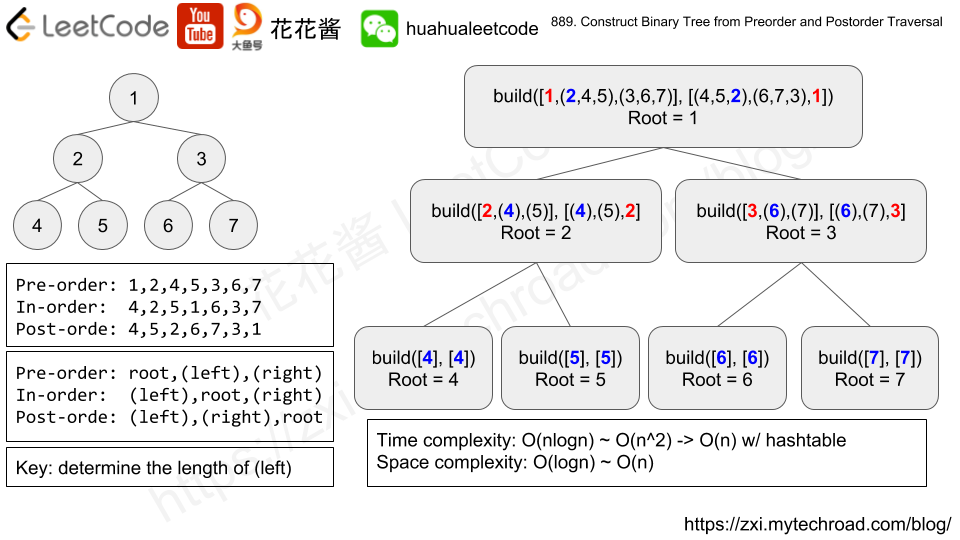# Posts tagged as “traversal”

Given a binary tree, return the preorder traversal of its nodes’ values.

Example:

Input: [1,null,2,3]
1
\
2
/
3

Output: [1,2,3]


Follow up: Recursive solution is trivial, could you do it iteratively?

## Solution 1: Recursion

Time complexity: O(n)
Space complexity: O(n)

## Solution 2: Stack

Time complexity: O(n)
Space complexity: O(n)

## C++

Given two binary search trees root1 and root2.

Return a list containing all the integers from both trees sorted in ascending order.

Example 1:

Input: root1 = [2,1,4], root2 = [1,0,3]
Output: [0,1,1,2,3,4]


Example 2:

Input: root1 = [0,-10,10], root2 = [5,1,7,0,2]
Output: [-10,0,0,1,2,5,7,10]


Example 3:

Input: root1 = [], root2 = [5,1,7,0,2]
Output: [0,1,2,5,7]


Example 4:

Input: root1 = [0,-10,10], root2 = []
Output: [-10,0,10]


Example 5:

Input: root1 = [1,null,8], root2 = [8,1]
Output: [1,1,8,8]


Constraints:

• Each tree has at most 5000 nodes.
• Each node’s value is between [-10^5, 10^5].

## Solution: Inorder traversal + Merge Sort

Time complexity: O(t1 + t2)
Space complexity: O(t1 + t2)

## C++/STL

Given a binary tree, return the vertical order traversal of its nodes values.

For each node at position (X, Y), its left and right children respectively will be at positions (X-1, Y-1) and (X+1, Y-1).

Running a vertical line from X = -infinity to X = +infinity, whenever the vertical line touches some nodes, we report the values of the nodes in order from top to bottom (decreasing Y coordinates).

If two nodes have the same position, then the value of the node that is reported first is the value that is smaller.

Return an list of non-empty reports in order of X coordinate.  Every report will have a list of values of nodes.

Example 1:

Input: [3,9,20,null,null,15,7]
Output: [,[3,15],,]
Explanation:
Without loss of generality, we can assume the root node is at position (0, 0):
Then, the node with value 9 occurs at position (-1, -1);
The nodes with values 3 and 15 occur at positions (0, 0) and (0, -2);
The node with value 20 occurs at position (1, -1);
The node with value 7 occurs at position (2, -2).


Example 2:

Input: [1,2,3,4,5,6,7]
Output: [,,[1,5,6],,]
Explanation:
The node with value 5 and the node with value 6 have the same position according to the given scheme.
However, in the report "[1,5,6]", the node value of 5 comes first since 5 is smaller than 6.


Note:

1. The tree will have between 1 and 1000 nodes.
2. Each node’s value will be between 0 and 1000.

## Solution: Ordered Map+ Ordered Set

Time complexity: O(nlogn)
Space complexity: O(n)

## Python3

Given a binary tree with N nodes, each node has a different value from {1, ..., N}.

A node in this binary tree can be flipped by swapping the left child and the right child of that node.

Consider the sequence of N values reported by a preorder traversal starting from the root.  Call such a sequence of N values the voyage of the tree.

(Recall that a preorder traversal of a node means we report the current node’s value, then preorder-traverse the left child, then preorder-traverse the right child.)

Our goal is to flip the least number of nodes in the tree so that the voyage of the tree matches the voyagewe are given.

If we can do so, then return a list of the values of all nodes flipped.  You may return the answer in any order.

If we cannot do so, then return the list [-1].

Example 1:

Input: root = [1,2], voyage = [2,1]
Output: [-1]


Example 2:

Input: root = [1,2,3], voyage = [1,3,2]
Output: 


Example 3:

Input: root = [1,2,3], voyage = [1,2,3]
Output: []


Note:

1. 1 <= N <= 100

## Solution: Pre-order traversal

if root->val != v[pos] return [-1]
if root->left?->val != v[pos + 1], swap the nodes

# Problem

Return any binary tree that matches the given preorder and postorder traversals.

Values in the traversals pre and post are distinct positive integers.

Example 1:

Input: pre = [1,2,4,5,3,6,7], post = [4,5,2,6,7,3,1]
Output: [1,2,3,4,5,6,7]


Note:

• 1 <= pre.length == post.length <= 30
• pre[] and post[] are both permutations of 1, 2, ..., pre.length.
• It is guaranteed an answer exists. If there exists multiple answers, you can return any of them.# Solution: Recursion

pre = [(root) (left-child) (right-child)]

post = [(left-child) (right-child) (root)]

We need to recursively find the first node in pre.left-child from post.left-child

e.g.

pre = [(1), (2,4,5), (3,6,7)]

post = [(4,5,2), (6,7,3), (1)]

First element of left-child is 2 and the length of it is 3.

root = new TreeNode(1)
root.left = build((2,4,5), (4,5,2))
root.right = build((3,6,7), (6,7,3))

Time complexity: O(n^2)

Space complexity: O(n)

Time complexity: O(n^2)

Space complexity: O(n)

Time complexity: O(n)

Mission News Theme by Compete Themes.